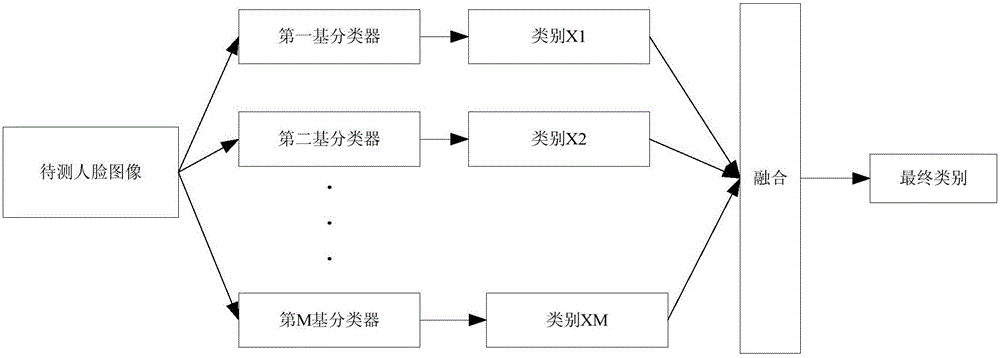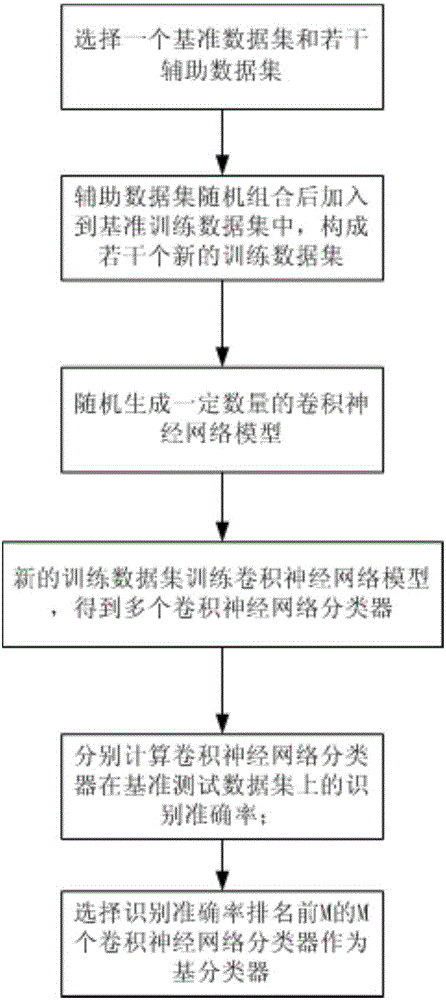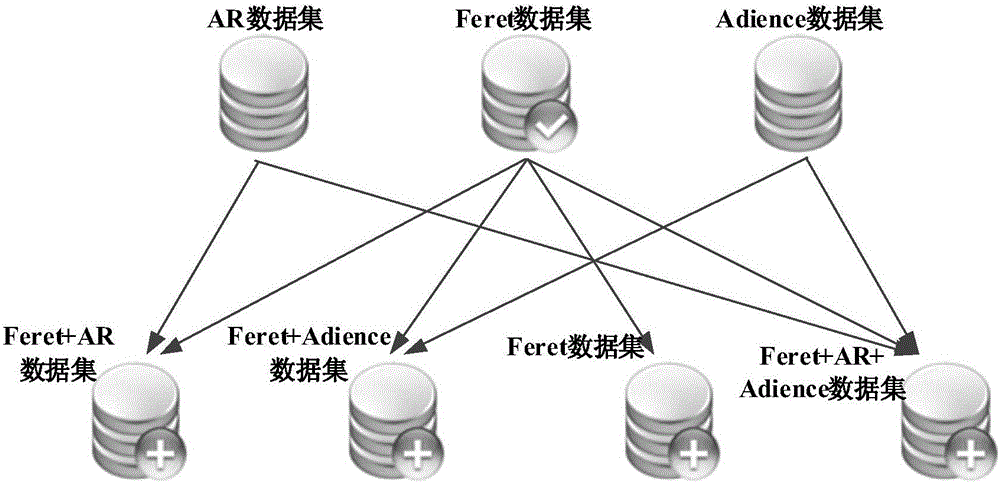# Integrated convolutional neural network-based gender recognition method

## A convolutional neural network and neural network technology, applied in character and pattern recognition, instruments, computer parts, etc., can solve the problems that affect the performance of face gender recognition, heavy workload, and complex parameter tuning, and achieve a good face. The effect of gender recognition performance, reducing dependencies, and improving accuracy

Active Publication Date: 2017-01-04
SOUTH CHINA UNIV OF TECH
2 Cites 16 Cited by

## AI-Extracted Technical Summary

### Problems solved by technology

This process relies heavily on the experience of human experts and repeated experiments. Not only is the workload heavy, but it is also difficult to find an optimal expression of facial gender characteristics, which affects the effect of facial gender recognition.
On the other hand, a sing...
View more

## Abstract

The invention discloses an integrated convolutional neural network-based gender recognition method. The method comprises the following steps: S1, first carrying out random combination to form a plurality of new training data sets, and selecting M convolutional neural network classifiers which are obtained through training by using the abovementioned new training data sets to serve as base classifiers; S2, obtaining a to-be-tested facial image; and S3, during the test, respectively inputting the to-be-tested facial image into the M base classifiers obtained in the step S1, and fusing gender types output by the M base classifiers to obtain a final gender type. According to the method disclosed by the invention, the convolutional neural network classifiers which are obtained through training by using the randomly obtained new training data sets serve as the base classifiers, the to-be-tested facial image is input into the M base classifiers, and finally the gender types output by the M base classifiers are fused to obtain the final gender type, so that the method has the advantages of being high in recognition accuracy rate, reducing the dependency, on the people, of the gender feature extraction of the facial image, and being wide in application.

Application Domain

Character and pattern recognition

Technology Topic

Training data setsSvm classifier +3

## Image

•••## Examples

• Experimental program(1)

### Example Embodiment

 Example
 This embodiment discloses a gender recognition method based on an integrated convolutional neural network, such as figure 1 As shown, the steps are as follows:
 S1. First, randomly combine to form a number of new training data sets, and then select M convolutional neural network classifiers trained on the above new training data sets as the base classifiers, which are the first base classifier and the second Base classifier,..., M-th base classifier; such as figure 2 As shown, the basic classifier acquisition process in this step is as follows:
 S11. Select a benchmark data set and several auxiliary data sets; wherein the benchmark data set is divided into a benchmark training data set and a benchmark test data set; image 3 As shown, in this embodiment, the Feret data set is selected as the benchmark data set, and the Adience data set and the AR data set are auxiliary data sets; the Feret data set is divided into a benchmark training data set and a benchmark test data set according to a ratio of 4:1;
 S12. The auxiliary data set is randomly combined and added to the reference training data set to form several new training data sets; in this embodiment, the auxiliary data set Adience data set and AR data set are added to the reference training data set of the Feret data set , Can form 4 new training data sets, namely Feret data set, Feret data set + Adience data set, Feret data set + AR data set, Feret data set + Adience data set + AR data set.
 S13. A certain number of convolutional neural network models are randomly generated; in the method of this embodiment, two convolutional neural network models are randomly generated, namely the five-layer convolutional layer convolutional neural network model G_CNNS_5 and the six-layer convolutional layer convolution Product neural network model G_CNNS_6.
 S14. Use several new training data sets obtained in step S12 to train the convolutional neural network model generated in step S13 respectively to obtain multiple convolutional neural network classifiers; in this embodiment, 4 obtained in step S12 Two data sets are trained on the two convolutional neural network models generated in step S13, and eight convolutional neural network classifiers are obtained through training; the classifier of the convolutional neural network classifier adopts the SoftMax classifier.
 S15. Calculate the recognition accuracy of each convolutional neural network classifier obtained in step S14 on the benchmark test data set; in this embodiment, calculate the 8 convolutional neural network classifiers obtained in step S14 in the Feret data set. The recognition accuracy rate on the benchmark data set.
 S16. Select M convolutional neural network classifiers with the top M recognition accuracy as base classifiers. In this embodiment, M is 5, that is, the top 5 convolutional neural network classifiers in the recognition accuracy of the 8 convolutional neural network classifiers in this step are selected as the base classifiers, and 5 base classifiers are obtained .
 S2, obtain a face image to be tested;
 S3. During the test, input the face image to be tested into the M base classifiers obtained in step S1, and obtain the output categories of the M base classifiers, which are category X1, category X2,..., category XM, and then merge them The gender categories output by the M base classifiers are finally one gender category.
 The network tuning parameter configuration of each convolutional neural network model randomly generated in this embodiment may be as shown in Table 1 below;
 Table 1
 Meta parameter Parameter value Remarks max_iter 50000 Training stops when the convolution iteration is set to 50000 times base_lr 0.001 Set the learning rate to 0.001 solver_mode GPU Set the training mode to use GPU for training weight_decay 0.0005 Set the weight decay rate to 0.0005 to prevent overfitting snapshot 10000 Set to save snapshots of prediction models, etc. every 10,000 times
 Such as Figure 4 As shown, the input layer to the output layer of the convolutional neural network model G_CNNS_6 of the six-layer convolutional layer in the above step S13 of this embodiment are sequentially connected with:
 The first data layer Date21 layer, this layer mainly sets the training set, defines that the input data adopts the data format of LMDB (memory mapped database), the uniform size of the picture is 227*227, the number of batch processing pictures is 128, and defines the input The path source of the training set.
 The second data layer is the Date22 layer, which sets the verification set and also uses the LMDB data format. The uniform size of the picture is 227*227, the number of batch processing pictures is 50, and the file directory source of the input verification set is configured.
 The first convolutional layer conv21 layer, the initial bias in this layer is 0, and the weight is calculated by a Gaussian filter with a variance of 0.01; the size of the convolution kernel of this layer is 11*11, and the number of convolution kernels is 96; after this layer The size of the feature map after convolution is 55*55; the learning rate of the convolution kernel of this layer is 1 and the attenuation factor is 1, the biased learning rate is 2 and the attenuation factor is 0.
 The first downsampling layer P21 layer, this layer uses the Max_pooling algorithm for downsampling, set the downsampling area size to 3*3, the pooling step size to 2, and the feature map size becomes 27*27 after downsampling.
 The first LRN (Local Response Normalization, that is, the local response normalization layer) layer is the L21 layer, and this layer uses the LRN for normalization. Using the default ACROSS_CHANNELS mode, the local area can be extended to adjacent channels for normalization, but the spatial range remains unchanged. Set the number of channels for summation local_size to 5, and the scale parameter alpha to 0.0001.
 The second convolutional layer conv22 layer, the size of the convolution kernel of this layer is 5*5, the number of convolution kernels is 256, and the edge supplement of the convolution kernel is 2; the group is set to 2 in this layer, which is the channel for input and output For grouping, the output channels can only be connected to the input channels of the same group.
 The second down-sampling layer P22 uses the Max_pooling algorithm for down-sampling. Set the down-sampling area size to 3*3, the pooling step size to 2, and the feature map size to 13*13 after down-sampling.
 The second LRN layer, L22 layer, uses LRN for normalization. The default ACROSS_CHANNELS mode is adopted, and the parameter values ​​are the same as the first LRN layer L21 layer.
 The third convolutional layer conv23, the size of the convolution kernel of this layer is 3*3, the number of convolution kernels is 384, and the edge supplement of the convolution kernel is 1; set the learning rate of the convolution kernel of this layer to 1 and the attenuation factor Is 1, the bias learning rate is 2 and the attenuation factor is 0.
 The fourth convolutional layer is conv24. The size of the convolution kernel of this layer is 3*3, the number of convolution kernels is 384, and the edge supplement of the convolution kernel is 1. Set group to 2 to group the input and output channels.
 The fifth convolutional layer conv25, the size of the convolution kernel of this layer is 3*3, the number of convolution kernels is 256, and the edge supplement of the convolution kernel is 1. Set group to 2 to group the input and output channels.
 The sixth convolutional layer conv26 layer, the size of the convolution kernel of this layer is 3*3, the number of convolution kernels is 256, and the edge supplement of the convolution kernel is 1; set group to 2 to group the input and output channels.
 The sixth downsampling layer P26 uses the Max_pooling algorithm for downsampling. The size of the downsampling area is 3*3, the pooling step size is 2, and the feature map size becomes 6*6 after downsampling.
 The first full link layer Q21 layer, output 4096 full connections.
 The first Dropout (random drop rate) layer D21 layer, the probability of the output value is set to 0, which can improve the overfitting of the training.
 The second full link layer Q22 layer, output 4096 full connections.
 And the second Dropout layer D12; set the output value to 0 with probability, which can improve the overfitting of training.
 Among them, the first conv layer conv21, the second conv layer conv22, the third conv layer conv23, the fourth conv layer conv24, the fifth conv layer conv25, the sixth conv layer conv26, the first A full link layer Q21 layer and a second full link layer Q22 layer correspond to the first activation function layer ReLU21 layer, the second activation function layer ReLU22 layer, the third activation function layer ReLU23 layer, and the fourth activation function layer ReLU24 layer, respectively. The fifth activation function layer ReLU25 layer, the sixth activation function layer ReLU26 layer, the seventh activation function layer ReLU27 layer, and the eighth activation function layer ReLU28 layer. First activation function layer ReLU21 layer, second activation function layer ReLU22 layer, third activation function layer ReLU23 layer, fourth activation function layer ReLU24 layer, fifth activation function layer ReLU25 layer, sixth activation function layer ReLU26 layer, seventh The activation function layer ReLU27 layer and the eighth activation function layer ReLU28 layer respectively use the ReLU (modified linear unit) activation function to activate the neurons of the connected layer.
 The structure difference between the five-layer convolutional layer convolutional neural network model G_CNNS_5 and the aforementioned six-layer convolutional layer convolutional neural network model G_CNNS_6 used in the above step S13 in this embodiment is only in the number of convolutional layers. The rest are the same. In this embodiment, the input layer to the output layer of the convolutional neural network model G_CNNS_5 of the five-layer convolutional layer are sequentially connected with the first data layer Date11 layer, the second data layer Date12 layer, the first convolution layer conv11 layer, and the first layer. Downsampling layer P11 layer, first LRN layer L11 layer, second convolutional layer conv12 layer, second downsampling layer P12 layer, second LRN layer L12 layer, third convolution layer conv13 layer, fourth convolution layer conv14 Layer, fifth convolutional layer conv15 layer, fifth down-sampling layer P15 layer, first full link layer Q11 layer, first Dropout layer D11 layer, second full link layer Q12 layer, and second Dropout layer D12 layer; Convolutional layer conv11, second conv12, third conv13, fourth conv14, fifth conv15, first full link layer Q11, second full link Layer Q12 is connected to the first activation function layer ReLU11 layer, the second activation function layer ReLU12 layer, the third activation function layer ReLU13 layer, the fourth activation function layer ReLU14 layer, the fifth activation function layer ReLU15 layer, and the sixth activation layer. The function layer ReLU16 layer and the seventh activation function layer ReLU17 layer. The parameters in each layer of the five-layer convolutional layer G_CNNS_5 and the six-layer convolutional layer G_CNNS_6 have the same parameters.
 In this embodiment, the five base classifiers selected in the above step S16 are the first base classifier CNN. 1 , The second base classifier CNN 2 , The third base classifier CNN 3 , The fourth base classifier CNN 4 And the fifth base classifier CNN 5; Among them, as shown in Table 2 below, the first base classifier CNN 1 The convolutional neural network model G_CNNS_6 of the six-layer convolutional layer is trained from the Feret data set, and the second base classifier CNN 2 The convolutional neural network model G_CNNS_5 of the five-layer convolutional layer trained from the Feret data set + AR data set, the third base classifier CNN 3 It is obtained by training the convolutional neural network model G_CNNS_6 of the six-layer convolutional layer from the Feret data set + AR data set, and the fourth base classifier CNN 4 From the Feret data set + Adience data set training five-layer convolutional layer convolutional neural network model G_CNNS_5, the fifth base classifier CNN 5 The five-layer convolutional layer convolutional neural network model G_CNNS_5 is obtained from the Feret data set + Adience data set + AR data set. In this embodiment, the face images to be tested are respectively input to the first base classifier CNN 1 , The second base classifier CNN 2 , The third base classifier CNN 3 , The fourth base classifier CNN 4 And the fifth base classifier CNN 5 Then, the simple voting fusion method is used to fuse the gender categories output by the five base classifiers, and the recognition results output by the five base classifiers are voted to obtain a final gender category, as shown in Table 2 below. The gender recognition accuracy rate of the gender recognition method in the embodiment can reach 97.82%.
 Table 2



## PUM## Description & Claims & Application Information

We can also present the details of the Description, Claims and Application information to help users get a comprehensive understanding of the technical details of the patent, such as background art, summary of invention, brief description of drawings, description of embodiments, and other original content. On the other hand, users can also determine the specific scope of protection of the technology through the list of claims; as well as understand the changes in the life cycle of the technology with the presentation of the patent timeline. Login to view more.

## Imaging apparatus and flicker detection method

ActiveUS20100013953A1reduce dependencyimprove accuracy
Owner:RENESAS ELECTRONICS CORP

## Techniques for sentiment analysis of data using a convolutional neural network and a co-occurrence network

ActiveUS20180341839A1improve accuracy
Owner:ORACLE INT CORP

## Color interpolation method

InactiveUS20050117040A1improve accuracy
Owner:MEGACHIPS

## Emotion classifying method fusing intrinsic feature and shallow feature

ActiveCN105824922AImprove classification performanceimprove accuracy
Owner:CHONGQING UNIV OF POSTS & TELECOMM

## Scene semantic segmentation method based on full convolution and long and short term memory units

InactiveCN107480726Aimprove accuracylow resolution accuracy
Owner:UNIV OF ELECTRONIC SCI & TECH OF CHINA

## Classification and recommendation of technical efficacy words

• improve accuracy
• reduce dependence

InactiveUS20050277485A1improve grip comfortimprove accuracy
Owner:FUSHENG IND CO LTD

## Stent delivery system with securement and deployment accuracy

ActiveUS7473271B2improve accuracyreduces occurrence and/or severity
Owner:BOSTON SCI SCIMED INC

## Method for improving an HS-DSCH transport format allocation

InactiveUS20060089104A1improve accuracyincrease benefit
Owner:NOKIA SOLUTIONS & NETWORKS OY

## Catheter systems

ActiveUS20120059255A1increase selectivityimprove accuracy
Owner:ST JUDE MEDICAL ATRIAL FIBRILLATION DIV

## Gaming Machine And Gaming System Using Chips

ActiveUS20090075725A1improve accuracy
Owner:UNIVERSAL ENTERTAINMENT CORP
Who we serve
• R&D Engineer
• R&D Manager
• IP Professional
Why Eureka